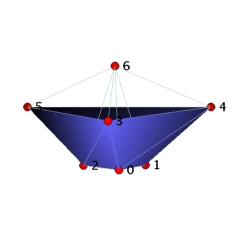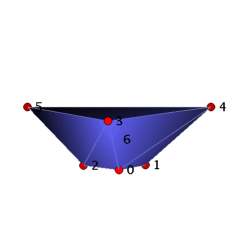Vertex-Minimal Not Vertex-Decomposable BallsElectronic Geometry Model No. 2003.06.001

Frank H. Lutz

Description

We present not vertex-decomposable simplicial d-balls with d+4 vertices for d≥3. Since it is known that d-balls with up to d+3 vertices are vertex-decomposable, these examples are vertex-minimal.

The concept of vertex-decomposability was introduced by Provan and Billera  who showed that all vertex-decomposable simplicial complexes, in particular, all vertex-decomposable simplicial spheres, satisfy the (simplicial form of the) famous Hirsch conjecture, which states that the diameter of the dual graph of a pure d-dimensional simplicial complex with n vertices is bounded above by n-(d+1). In fact, the original Hirsch conjecture, formulated by Hirsch in 1957 (cf. [3, p. 168]), plays an important role in the study of the computational complexity of the simplex algorithm of linear programming (see the surveys in  and ); it asserts that the diameter of the graph of a (d+1)-polytope with n facets, in other words, the number of pivot steps that an edge-following LP algorithm needs for this polytope in the worst case with respect to a best possible choice of the pivots, is smaller or equal to n-(d+1).

A pure d-dimensional simplicial complex K is vertex-decomposable if either K is a simplex (possibly the set containing the empty set) or there is a vertex v such that both the deletion of v in K, i.e., the set del_K(v) of faces of K that do not contain the vertex v, and the link of v in K, i.e., the set link_K(v) of faces of K that do not contain the vertex v, but for which their union with {v} is still in K, are vertex-decomposable pure simplicial complexes.

Provan and Billera  proved that triangulated 2-dimensional balls and spheres are vertex-decomposable and thus satisfy the Hirsch conjecture. Klee and Kleinschmidt further showed  that all simplicial d-balls (respectively d-spheres) with up to d+3 (respectively d+4) vertices are vertex-decomposable. However, Lockeberg  gave a simplicial 4-polytope with 12 vertices whose boundary sphere is not vertex-decomposable, and there even are not vertex-decomposable simplicial 4-polytopes with 10 vertices . The Hirsch conjecture for simplicial spheres was disproved in 1978 by Walkup  who provided a 27-dimensional counterexample with 56 vertices. A much smaller counterexample of dimension 11 with 24 vertices is due to Mani and Walkup . Nevertheless, the Hirsch conjecture for (simplicial) d-polytopes is still open for d≥4.

If a simplicial 3-sphere is not vertex-decomposable, then the deletion of any of its vertices yields a not vertex-decomposable simplicial 3-ball. We enumerated in  all triangulated 3-spheres (respectively 3-balls) with n≤10 (respectively n≤9) vertices and tested whether they are vertex-decomposable.

Theorem: There are precisely two vertex-minimal not vertex-decomposable triangulated 3-balls B_3_7_10 and B_3_7_11 with 7 vertices that have 10 and 11 facets, respectively.

It also follows from the enumeration in  that all simplicial 3-spheres with n≤8 vertices are vertex-decomposable, but that there are not vertex-decomposable (non-polytopal) simplicial 3-spheres with 9 vertices. The cone over a simplicial d-ball K with respect to a new vertex is a (d+1)-dimensional ball and is vertex-decomposable if and only if K is vertex-decomposable (cf. ). A similar statement holds for one-point suspensions of simplicial spheres; see .

Corollary: There are vertex-minimal not vertex-decomposable simplicial d-balls with d+4 vertices and 10 facets for d≥3. Moreover, there are not vertex-decomposable simplicial d-spheres with d+6 vertices for d≥3.

This corollary settles, at least for d-balls, a problem of Klee and Kleinschmidt [5, p. 740] who wondered how far the above bounds d+3 and d+4 for d-balls and d-spheres ``can be raised without losing vertex-decomposability''.

The ball B_3_7_10 can be visualized as a straight, convex ball with a Z_3 rotation symmetry as follows. We start with a ring of three tetrahedra 0134, 0235, 1245 and close the hole of the ring by gluing in the triangle 012. Then we place vertex 6 ``above'' the seven triangles 012, 013, 025, 035, 124, 134, 245, and we add the cone over these triangles with apex 6 to the complex. The resulting 3-ball with 7 vertices and 10 facets is not vertex-decomposable, e.g. the deletion of vertex 6 yields the base ring with glued in triangle 012 and hence is not pure. The ball B_3_7_11 can be obtained from B_3_7_10 by adding the tetrahedron 3456, for which vertex 6 has to be placed within the convex hull of the vertices 0-5.

We remark that vertex-decomposable simplicial complexes are shellable. For surveys on shellability, in particular, of balls and spheres, see , , and [13, Ch. 8]. A vertex-minimal non-shellable 3-ball with 9 vertices and 18 facets is described in . The smallest known non-shellable 3-sphere with 13 vertices and 56 facets can be found in .

 Keywords simplicial balls and spheres; vertex-decomposability; shellability; Hirsch conjecture MSC-2000 Classification 52B05 (90C60, 52B22, 57Q15) Zentralblatt No. 05264897

References

1. A. Björner: Topological methods, in R. Graham, M. Grötschel, and L. Lovász (Eds.): Handbook of Combinatorics, Elsevier (1995), 1819-1872.
2. J. Bokowski, D. Bremner, F. H. Lutz, and A. Martin: Combinatorial 3-manifolds with 10 vertices, in preparation.
3. G. B. Dantzig: Linear Programming and Extensions, Princeton University Press (1963).
4. M. Joswig and F. H. Lutz: One-point suspensions and wreath products of polytopes and spheres, in preparation.
5. V. Klee and P. Kleinschmidt: The d-step conjecture and its relatives, Math. Oper. Res. 12 (1987), 718-755.
6. E. R. Lockeberg: Refinements in Boundary Complexes of Polytopes, Ph.D. Thesis, University College London (1977).
7. F. H. Lutz: A vertex-minimal non-shellable simplicial 3-ball with 9 vertices and 18 facets, Electronic Geometry Models (2004), 2003.05.004, http://www.eg-models.de/2003.05.004.
8. F. H. Lutz: Small examples of non-constructible simplicial balls and spheres, SIAM J. Discrete Math., to appear.
9. P. Mani and D. W. Walkup: A 3-sphere counterexample to the W_v-path conjecture, Math. Oper. Res. 5 (1980), 595-598.
10. J. S. Provan and L. J. Billera: Decompositions of simplicial complexes related to diameters of convex polyhedra, Math. Oper. Res. 5 (1980), 576-594.
11. D. W. Walkup: The Hirsch conjecture fails for triangulated 27-spheres, Math. Oper. Res. 3 (1978), 224-230.
12. G. M. Ziegler: Shelling polyhedral 3-balls and 4-polytopes, Discrete Comput. Geom. 19 (1998), 159-174.
13. G. M. Ziegler: Lectures on Polytopes, Springer-Verlag, Graduate Texts in Mathematics 152 (1995; revised edition, 1998).

Submission information

Submitted: Sun Jun 1 12:11:52 GMT 2003.
Revised: Tue Mar 16 16:29:15 CET 2004.
Accepted: Sun Mar 21 11:07:26 CET 2004.